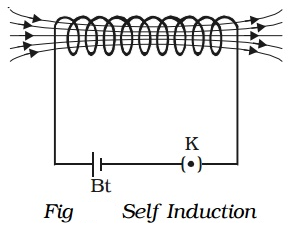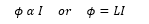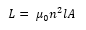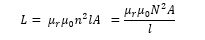The property of a coil which enables to produce an opposing induced emf in it when the current in the coil changes is called self induction.A coil is connected in series with a battery and a key (K) (Fig.). On pressing the key, the current through the coil increases to a maximum value and correspondingly the magnetic flux linked with the coil also increases. An induced current flows through the coil which according to Lenz’s law opposes the further growth of current in the coil.

On releasing the key, the current through the coil decreases to a zero value and the magnetic flux linked with the coil also decreases. According to Lenz’s law, the induced current will oppose the decay of current in the coil.

### Coefficient of self induction:

When a current I flows through a coil, the magnetic flux (φ) linked with the coil is proportional to the current.where L is a constant for the given coil and is called self inductance or coefficient of self induction of the coil.

Any change in current sets up an induced emf in the coil given byCase1: If I = 1 , then ϕ = L

Thus the self inductance of a coil is numerically equal to the magnetic flux linked with the coil when a unit current flows through it.

Case 2: If dI/dt = 1, then e = -L

Thus the self inductance of a coil may be defined as the induced emf set up in the coil due to a unit rate of change of courrent through it.

Unit of self inductance: 1henry (H)

### Self-inductance of a long solenoid:If N is the total number of turns in the solenoid, then n = N/l and so,If the coil is wound over a material of high relative magnetic permeability (e.g., soft iron), then